Full Text:<3143>

Summary:<1912>

CLC number: V21

On-line Access: 2014-03-04

Revision Accepted: 2014-01-17

Crosschecked: 2014-02-20

Cited: 5

Clicked: 6512

Citations:  Bibtex RefMan EndNote GB/T7714

 Journal of Zhejiang University SCIENCE A 2014 Vol.15 No.3 P.185-196 http://doi.org/10.1631/jzus.A1300341Numerical simulation of aerodynamic heating and stresses of chemical vapor deposition ZnS for hypersonic vehicles*

 Author(s):  Yuan-chun Liu1, Yu-rong He1, Jia-qi Zhu2, Jie-cai Han2, Dong-liang Quan3 Affiliation(s):  1. School of Energy Science & Engineering, Harbin Institute of Technology, Harbin 150001, China; more Corresponding email(s):   rong@hit.edu.cn Key Words:  Chemical vapor deposition (CVD) ZnS, Infrared window material, Thermal and stress responses, Hypersonic vehicles Share this article to： More <<< Previous Article|Next Article >>>

Yuan-chun Liu, Yu-rong He, Jia-qi Zhu, Jie-cai Han, Dong-liang Quan. Numerical simulation of aerodynamic heating and stresses of chemical vapor deposition ZnS for hypersonic vehicles[J]. Journal of Zhejiang University Science A, 2014, 15(3): 185-196.

@article{title="Numerical simulation of aerodynamic heating and stresses of chemical vapor deposition ZnS for hypersonic vehicles",
author="Yuan-chun Liu, Yu-rong He, Jia-qi Zhu, Jie-cai Han, Dong-liang Quan",
journal="Journal of Zhejiang University Science A",
volume="15",
number="3",
pages="185-196",
year="2014",
publisher="Zhejiang University Press & Springer",
doi="10.1631/jzus.A1300341"
}

%0 Journal Article
%T Numerical simulation of aerodynamic heating and stresses of chemical vapor deposition ZnS for hypersonic vehicles
%A Yuan-chun Liu
%A Yu-rong He
%A Jia-qi Zhu
%A Jie-cai Han
%A Dong-liang Quan
%J Journal of Zhejiang University SCIENCE A
%V 15
%N 3
%P 185-196
%@ 1673-565X
%D 2014
%I Zhejiang University Press & Springer
%DOI 10.1631/jzus.A1300341

TY - JOUR
T1 - Numerical simulation of aerodynamic heating and stresses of chemical vapor deposition ZnS for hypersonic vehicles
A1 - Yuan-chun Liu
A1 - Yu-rong He
A1 - Jia-qi Zhu
A1 - Jie-cai Han
A1 - Dong-liang Quan
J0 - Journal of Zhejiang University Science A
VL - 15
IS - 3
SP - 185
EP - 196
%@ 1673-565X
Y1 - 2014
PB - Zhejiang University Press & Springer
ER -
DOI - 10.1631/jzus.A1300341

Abstract:
hypersonic vehicles subjected to strong aerodynamic forces and serious aerodynamic heating require more stringent design for an infrared window. In this paper, a finite element analysis is used to present the distributions of thermal and stress fields in the infrared window for hypersonic vehicles based on flowfield studies. A theoretical guidance is provided to evaluate the influence of aerodynamic heating and forces on infrared window materials. The aerodynamic heat flux from Mach 3 to Mach 6 flight at an altitude of 15 km in a standard atmosphere is obtained through flowfield analysis. The thermal and stress responses are then investigated under constant heat transfer coefficient boundary conditions for different Mach numbers. The numerical results show that the maximum stress is higher than the material strength at Mach 6, which means a failure of the material may occur. The maximum stress and temperatures are lower than the material strength and melting point under other conditions, so the material is safe.

## 1.  Introduction

Aircraft and spacecraft structures designed for supersonic and hypersonic flights are subjected to severe aerodynamic heating during the launch and reentry phases of their operations, which is caused by the air in the boundary layer being progressively slowed down (Ruan et al., ). Consequently, all external surfaces on the vehicle are heated. This in turn leads to non-uniform transient temperatures that produce dynamic thermal stresses and deformations. Therefore, high heating associated with shocks at leading edges are significant issues in the vehicle design. In addition to surface melting and ablation, the flight aerodynamics can be perturbed, leading to unacceptable flight trajectory deviations. Another problem is signal refraction passing through the shocked hot gas layer in front of the vehicle’s head (Saravanan et al., ). In recent years, there have been significant investments in the development of hypersonic vehicle technologies. Hypersonic flight began in February 1949 when a Women’s Army Corps (WAC) corporal rocket was ignited from a US captured V-2 rocket (Sun and Wu, ). Later, extensive numerical analysis (Jain and Hayes, ; Di Clemente et al., ; Gerdroodbary and Hosseinalipour, ) obtained the pressure, heat transfer, and surface temperatures on steady or unsteady heat transfer in boundary layers of hypersonic flow. Although some flight experiments are also conducted (Di Clemente et al., ; Marini et al., ) to acquire aerodynamic heating data in the flight condition to evaluate these prediction methods, flight data are not adequate for complete validations. The coupled approach between the external and internal fields in hypersonic conditions has been studied in recent years (Culler and McNamara, ). Di Clemente et al. () studied the surface temperature measurements in a plasma wind tunnel experiment and compared these results with the results of the test numerical rebuilding carried out through an integrated procedure coupling the external aerodynamic field to the internal thermal state of the structure.

As one of the key components of a hypersonic vehicle, an infrared window is used to transmit infrared signals, and to keep the aerodynamic shape and protect its imaging system. High levels of aerodynamic heating can cause a malfunction or even damage to the delicate onboard equipment (White, ; Gnemmi et al., ). Excessive heating can cause ablation to the vehicle surface material. Combined with the presence of high-pressure loads, severe heating can cause a complete material failure and affect the normal performance of other instruments underneath the window (Heubner et al., ). The window design requires (Russell et al., ): (1) accommodating the severe highly transient aeroheating and resulting thermal gradients; (2) providing an effective seal to prevent gas flow into the laser guidance electronics volume; (3) minimizing aerodynamic loads by surface flush; (4) minimizing dynamic effects on the window due to launch and flight shocks; (5) providing an adequate surface finish and transmissibility for the laser wavelength of interest.

Zinc sulphide (ZnS) is one of the most common materials for infrared windows (Harris et al., ). The most common technique to deposit cubic polycrystalline ZnS thick films for optical window applications is based on a chemical vapor deposition (CVD) process (Harris, ). This technique is faster and cheaper than the epitaxial methods (molecular beam epitaxy (MBE) and atomic layer epitaxy (ALE)), and has better purity than the wet chemical method (Tropf et al., ).

The main objective of this paper is to present a theoretical guidance for the design of the infrared window (CVD ZnS) for hypersonic vehicles. To accomplish this, a process for predicting the aeroheating and thermal response of typical vehicle configurations in high-speed flows is described first. The aerodynamic heat flux from Mach 3 to Mach 6 at an altitude of 15 km in a standard atmosphere is obtained through flowfield analysis. Then, a finite element analysis using a coupling method is described to study the distribution of thermal and stress fields of the infrared window for hypersonic vehicles. The remainder of this paper is arranged as follows. In the next section, the mathematical model used is illustrated including the flowfield model, the structural model, and the Von Mises theory. Next, the main results of the simulation study are discussed. The paper finalizes with the main conclusions and recommendations for future studies.

## 2.  Mathematical model

### 2.1.1.  Basic physical models for fluid flow

For all flows, the conservation equations for mass and momentum are solved. For flows involving heat transfer or compressibility, an additional equation for energy conservation is solved. Additional transport equations are also solved when the flow is turbulent.

The equation for conservation of mass, or continuity equation, can be written as follows (Shahmardan et al., ; Tang et al., ): , where ρ is the density, t is the time, and v is the velocity.

For 2D geometries, the continuity equation is given by .

The conservation of momentum in an inertial (non-accelerating) reference frame is described by , where p is the pressure, g is the gravitational acceleration (=9.80665 m/s2), and F is the force vector. The stress tensor is given by , where μ is the molecular viscosity, and I is the unit tensor.

For 2D geometries, the axial and radial momentum conservation equations are given by where .

The energy equation for 2D geometries described by temperature can be written as follows: , where C p is the heat capacity at a constant pressure, T is the temperature, and λ is the thermal conductivity.

The continuity equation, momentum conservation equation, and energy equation can be described by the general differential equation: , where ϕ is the general variable, and stands for the solving variables, such as vx , vy , and T. Γϕ is the diffusion coefficient for ϕ, and Sϕ is the source of ϕ.

For each control volume V, the discretization of the general differential equation can be written as follows: , where A is the surface area vector.

For 2D geometries, the discretization for the cell face f on a given cell yields is , where N faces is the number of faces enclosing cell.

### 2.1.2.  Turbulence model

The shear-stress transport (SST) k-ω model was developed by Menter () to effectively blend the robust and accurate formulation of the k-ω model in the near-wall region with the free-stream independence of the k-ω model in the far field.

The SST k-ω model has a similar form to the standard k-ω model. The turbulence kinetic energy, k, and the specific dissipation rate, ω, are obtained from the following transport equations:

The effective diffusivities for the SST k-ω model are given by where σk is the turbulent Prandtl numbers for k, σω is the turbulent Prandtl numbers for ω, and μ t is the turbulent viscosity.

The term represents the production of turbulence kinetic energy, and is defined as , where , where is the compressibility function, and .

The term represents the production of ω, which is given by , where ι is a coefficient, in the high-Reynolds number form, ι=1, and ν t is the turbulent kinematic viscosity.

The term Yk represents the dissipation of turbulence kinetic energy, .

The term Yω represents the dissipation of ω, , where , .

The introduction of a cross-diffusion term Dω is defined as , where F 1 is the blending function, and .

### 2.2.  Structural model

2D geometries are used for the axisymmetric hypersonic flow in the fluid model. Therefore, the schematic quarter 3D model is used in the structural model to predict the temperature and stress distribution.

Based on the principle of energy balance, the solid wall temperature can be described by a differential relation. In a Cartesian coordinate system, the unsteady heat conduction differential equation without internal heat source is expressed as .

The boundary condition on the internal surface is adiabatic, while the boundary condition on the external surface is set as a constant thermal flux expressed as , where is the normal temperature gradient of a solid surface, h is the heat transfer coefficient, T a is the ambient temperature, and T s is the temperature of the window.

For the finite element analysis, the functional equation established by the weighted residual quantity is , where , ϖ is weight function, V is the volume of the element, and S is the boundary of the element.

Transforming the formula by Gauss-Green, integration is obtained as .

The discretization of the integral domain into elements and the temperature elements are interpolated as follows: , where N is the shape function matrix.

Introducing the boundary condition to the functionality, we can obtain: , where , where η and T b are known quantities; for boundary elements, ; for internal elements, .

The aerodynamic heating on the surface of the window is from the flowfield.

Meanwhile, the heat radiated into the low temperature space from the solid wall is ignored because it has little effect on the overall heat transfer for a relatively small Mach number.

There are two basic conditions for thermal stresses: temperature change and constraint. According to the Newton motion law, the aerospace mechanics balance equation for a 3D model is expressed as follows: where σxx , σyy , and σzz are the normal stresses, τxy , τyx , τxz , τzx , τyz , and τzy are the shearing stresses, fx , fy , and fz are the components of the body force, ux , uy , and uz are the displacements, and are dynamic terms.

The physical equation is given by (Ying et al., ): , where ε is the linear strain, γ is the shear strain, A 1=ψ/(1–ψ), A 2=(1–2ψ)/[2(1–ψ)], ψ is the Poisson’s ratio, E is the Young’s modulus, α is the thermal expansion, and ΔT is the increment of temperature.

The strain compatibility equations are expressed as (Ma et al., ) where the linear strain and the shear strain are defined as follows:

According to the Von Mises’s criterion, failure occurs when the strain energy of the distortion reaches a critical value. In this case, the equivalent stress σ eq is defined as (Carrera and Giunta, ; Jin et al., ) , where [σ] is the permissible stress.

### 2.3.  Physical model

To generalize the study of window structures, a hypersonic vehicle configuration is used in a hypersonic flow, as shown in Fig. 1. The overall length of the configuration is 3 m and it consists of two main sections: the nose and guidance section and the motor section. The nose and guidance section is a wedge-shaped body, such that the window located on the surface is inclined with respect to the freestream flow. The half-angle of the nose is 16°, with the distance from the nose tip being 1.046 m. The simulations are carried out in the Fluent 14.0® environment by a finite volume method.

Fig. 2 shows the schematic quarter model used in the simulation with symmetry conditions imposed on the two middle faces. The outermost is a titanium constraint frame to support the window with Grafoil™ at the interface between the frame and the window to provide a seal and minimize stresses. The innermost is the infrared functional window CVD ZnS material. The structural dimensions are shown in Fig. 3. A finite element analysis by ANSYS 14.0® is presented to study the distribution of thermal and stress fields using the coupled cell Solid 5.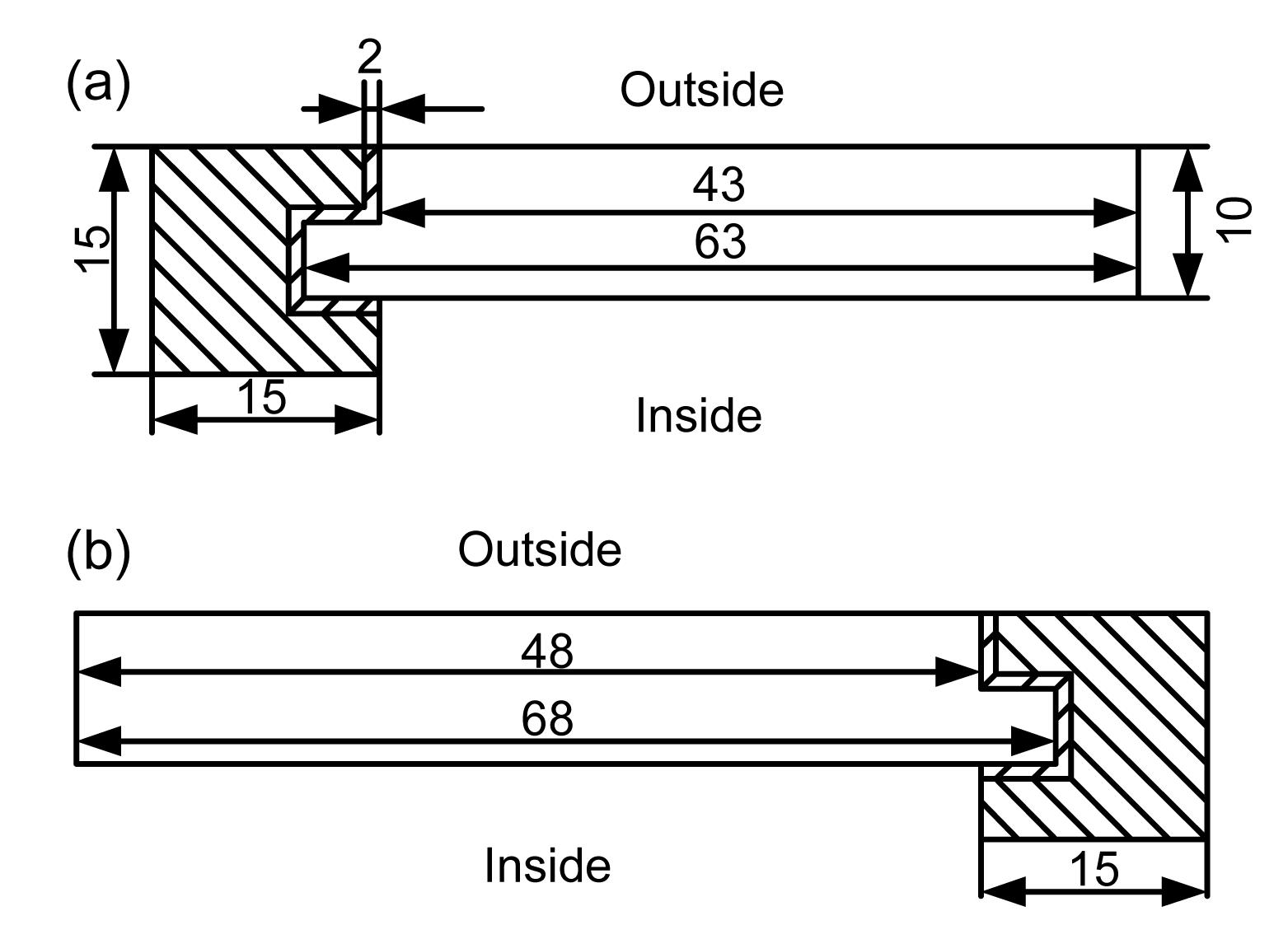Fig.3
Structural dimensions of window at XY plane (a) and YZ plane (b) (unit: mm)

The thermal and mechanical properties of the window are listed in Table 1. The Grafoil™ and CVD ZnS properties were taken from vendor data.

#### Table 1

Thermal and mechanical properties
 Material Density (kg/m3) Thermal conductivity (W/(m·K)) Specific heat (J/(kg·K)) Thermal expansion (1/K) Young’s modulus (GPa) Poisson’s ratio Mean strength (MPa) Titanium 4510 15.24 520 10.0×10−6 102.01 0.30 650 Grafoil™ 1121 5.19 712 2.7×10−5 0.20 0.30 0.7 CVD ZnS 4090 27.20 515 6.5×10−6 74.50 0.28 93

### 2.4.  Mesh generation

The flowfield model uses quadrilateral elements which are refined in the boundary layer and the number of grids on the X and Y directions are 1406 and 199, as shown in Fig. 4a. An implicit formulation is constructed at all grid points. Gradient is discretized using a Green-Gauss node based scheme. Concerning the flow, the turbulent kinetic energy and a specific dissipation rate are discretized using a second-order upwind scheme. The convergence precisions for the flowfield model are set to 1×10−5.Fig.4
Discretization of the computational domain for flowfield model (a) and structural model (b)

The structural model consists of thermal and stress fields using hexahedron elements with an initial temperature of 298 K and the number of grids for the structural model on X, Y, and Z directions are 110, 77, and 29, respectively (Fig. 4b). The preconditioned conjugate gradient (PCG) solver is applied for simulating the finite element model, and the convergence precisions for the structural model are set to 1×10−8.

The initial conditions used in fluid models are constant Mach numbers from 3 to 6 flight at an altitude of 15 km in a standard atmosphere. Consequently, the freestream flow conditions are P =12 112.0 Pa and T =216.8 K, and the wall temperature is 298 K.

Aerodynamic heating loads from the fluid models are applied to the skin of the structural models using a convection heating boundary condition, where the convection heat transfer coefficient on the outer surface and the adiabatic wall temperature on the inner surface are the inputs. The window is initially stress-free and has a uniform temperature of 298 K.

## 3.  Results and discussion

### 3.1.1.  Aerodynamic heating model verification

To validate the numerical solver, a case from the experimental investigation by Muylaert et al. () is selected. In the experiment, the surface heat flux referred to as ELECTRE was measured. The freestream flow conditions (293 s) were v =4230.0 m/s, p =53.0 Pa, and T =265.0 K. To test the grid sensitivity, three grids with different resolutions were examined prior to validating their results. The coarse, medium, and fine grids contained 5.160, 1.650, and 0.451 y plus, respectively. The profiles of heat flux variation along the body surface are plotted in Fig. 5. It is shown that the numerical solution based on the fine grid produces heat flux predictions that agree very well with experimental data.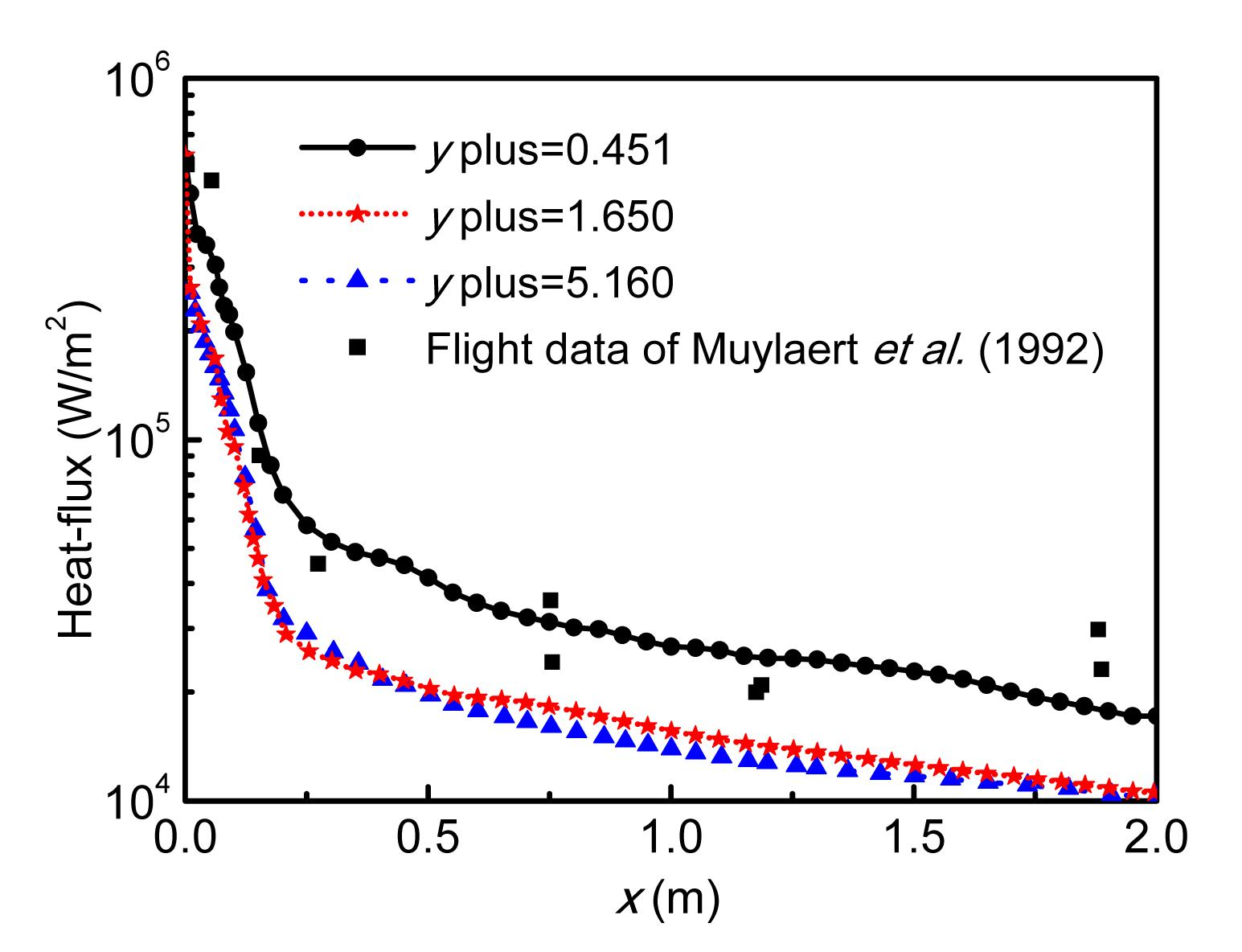Fig.5
Comparison of heat flux predictions between the propose method and Muylaert et al. ()

### 3.1.2.  Aerodynamic heating studies

Temperature contours of Mach 3, Mach 4, Mach 5, and Mach 6 are shown in Figs. 6a, 6b, 6c and 6d, respectively. Obviously, there is an attached oblique shock at the forebody leading edge at the hypersonic flow. The temperature indicates a large variation in the shock, especially near the solid wall. The maximum heating for all the Mach numbers occurs at the tip of nose (stagnation point) where the flow is usually decelerated and brings it down to zero velocity. In this region, boundary layers are very thin. In addition, the flowfield temperature increases with the increasing Mach number.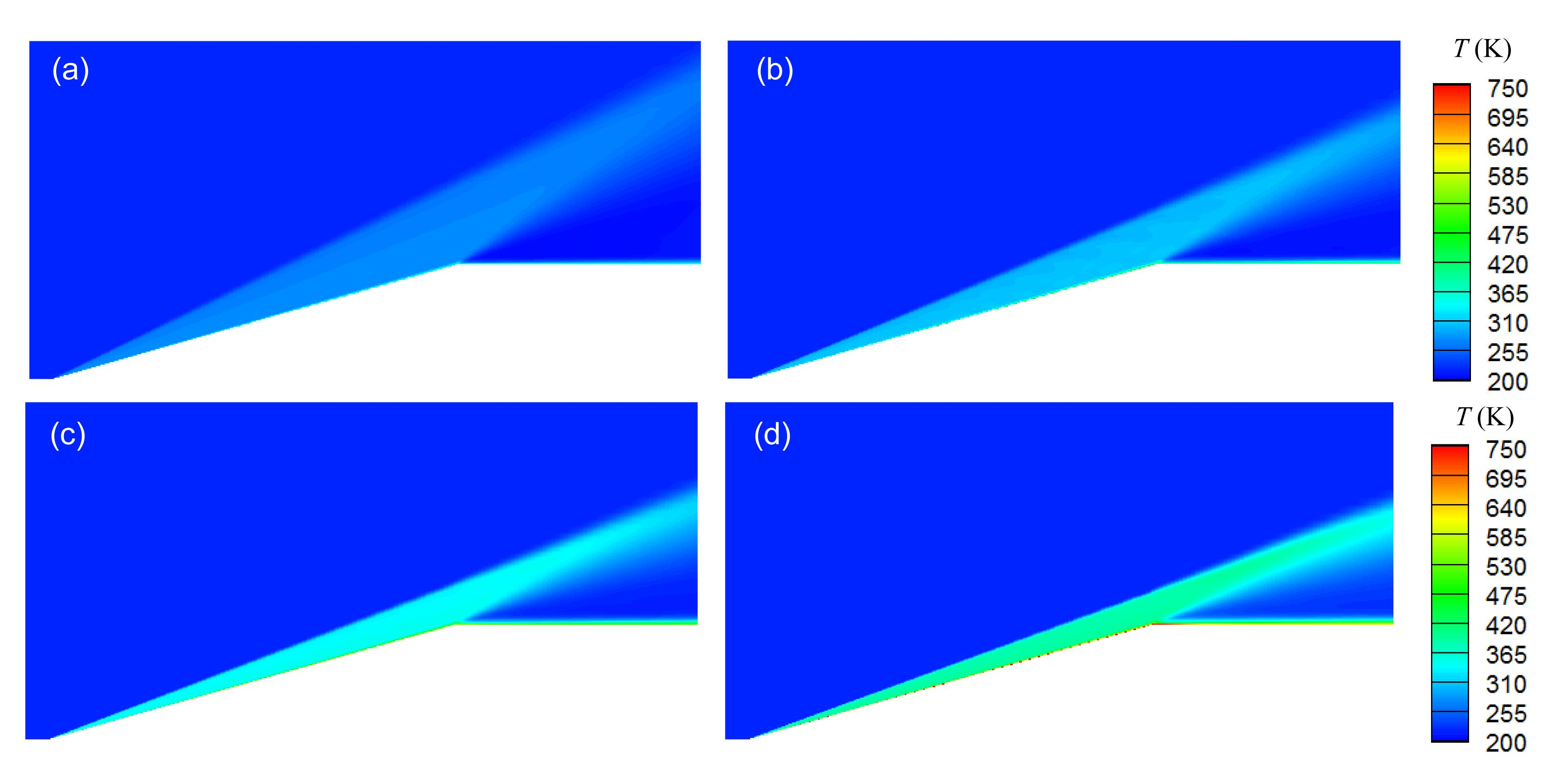Fig.6
Temperature contours of the numerical flowfield at Mach 3 (a), Mach 4 (b), Mach 5 (c), and Mach 6 (d)

The surface heat flux distributions along the body surface at Mach 3, Mach 4, Mach 5, and Mach 6 are shown in Fig. 7. For a constant Mach number, the maximum heat flux occurs near the stagnation zone of the model, and beyond the maximum heating point (stagnation point), the heat flux gradually decreases. After the axial location of 0.2 m (i.e., from the stagnation point), the heat flux is nearly constant, which is clearly seen in Fig. 7. For different Mach numbers, the heat flux increases gradually from Mach 3 to Mach 6. The location of the window is 0.55 m from the nose tip, where it reaches the minimum heat flux value. The heat transfer coefficient and ambient temperature at the window location at different Mach numbers are listed in Table 2.Fig.7
Heat flux along the body surface at different Mach numbers

#### Table 2

Value of heat transfer coefficient on the point that the window located
 Mach number Heat transfer coefficient (W/(m2·K)) Ambient temperature (K) 3 3084.57 361.78 4 7940.36 458.74 5 14848.22 605.65 6 23440.18 815.18

### 3.2.1.  Aerothermoelastic model verification

To determine the accuracy of the proposed method, the results are compared with those from Russell et al. (). From Fig. 8, we can see that the temperature is in good agreement with Russell et al. (). Therefore, the following work will be done using the proposed method.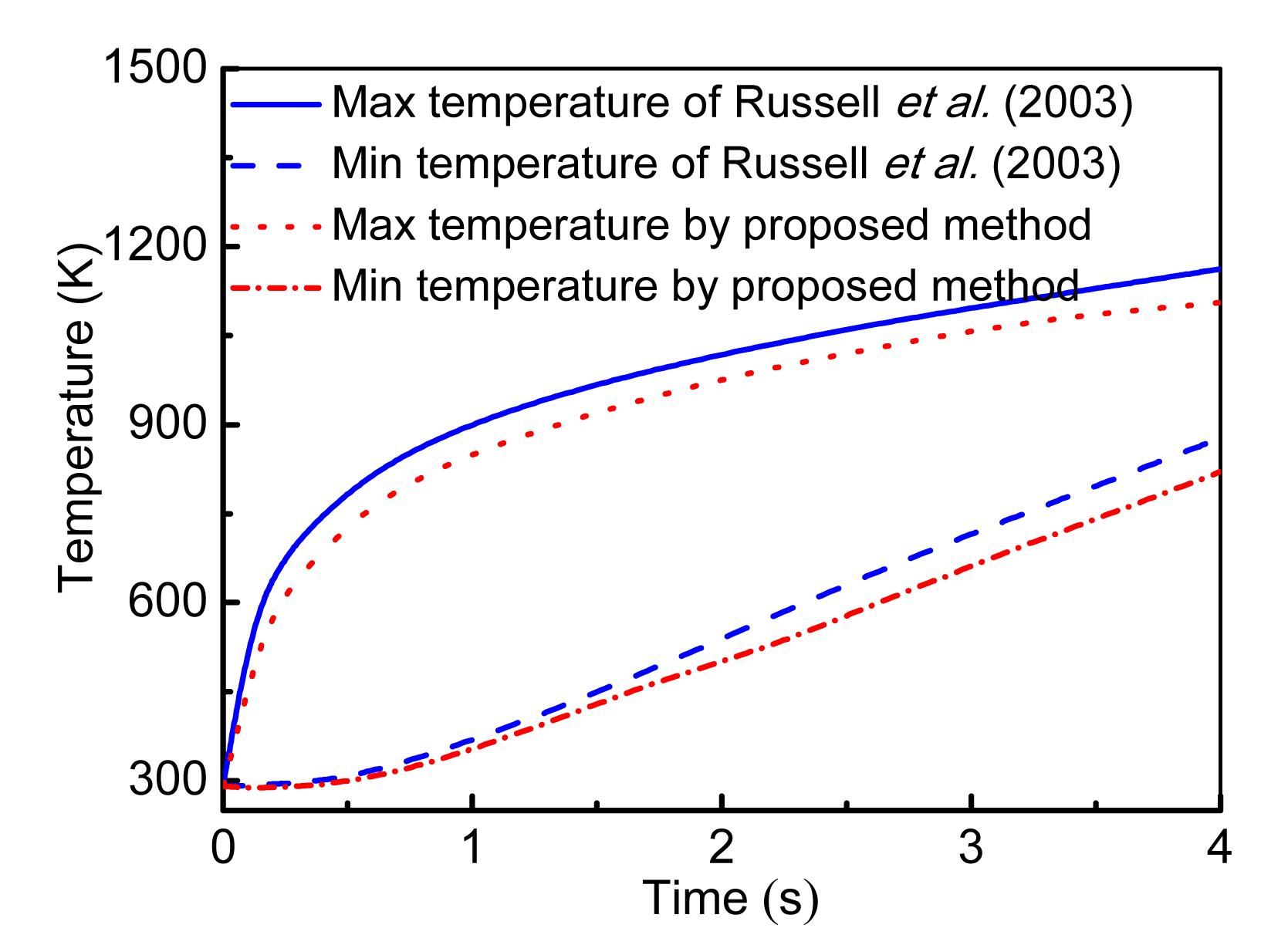Fig.8
Temperature results comparison between the proposed method and Russell et al. ()

### 3.2.2.  Aerothermoelastic studies

To determine the dangerous temperature and thermal stress that is likely to lead to a failure of infrared window, the heating loads from the fluid models (Table 2) are applied to the surface of the window to simulate the temperature and stress response at 10 s.

The thermal and stress responses at Mach 3, Mach 4, Mach 5, and Mach 6 are shown in Figs. 9 and 10, respectively.

Fig. 9 shows that the maximum temperature occurs at the center of the outer surface and the minimum occurs at the surrounding of the inner surface. The temperature decreases from inside to outside and from center to surrounding. It is caused by the severe aerothermal load on the outer surface of the window.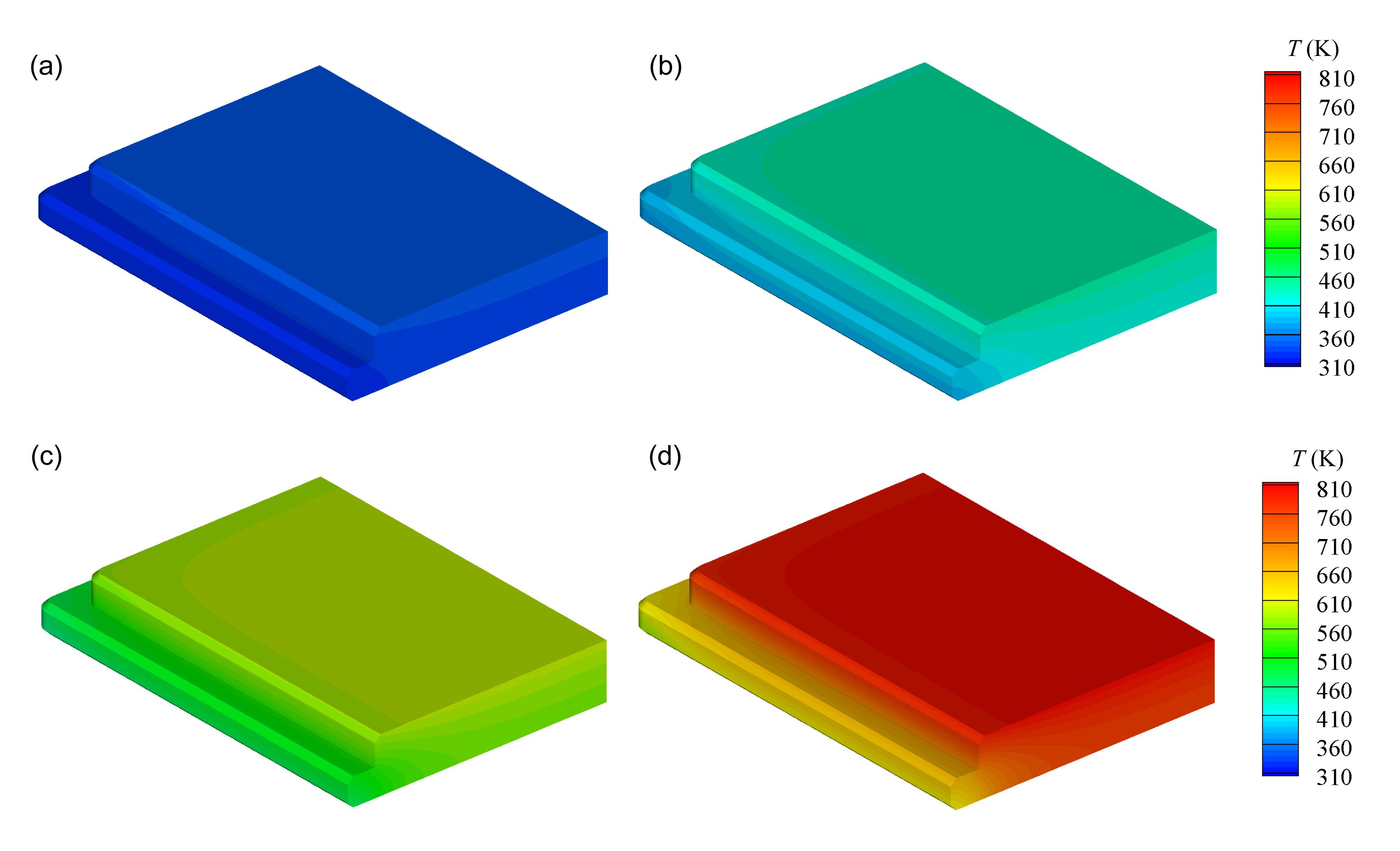Fig.9
Thermal response at 10 s at Mach 3 (a), Mach 4 (b), Mach 5 (c), and Mach 6 (d)

Fig. 10 shows that the stress at the step corner is high and the stress at the center of the outer surface is low. There also is a stress concentration on the step corner where the dangerous point of the window material lies.Fig.10
Stress response at 10 s at Mach 3 (a), Mach 4 (b), Mach 5 (c), and Mach 6 (d)

Distributions of maximum temperature, minimum temperature, and maximum stress at different conditions with time are shown in Figs. 11 and 12.

Fig. 11 shows the distributions of maximum and minimum temperatures with time. As illustrated in Fig. 11, the temperature increases with increasing Mach number. At the same Mach number, the maximum temperature which occurs at the outer surface increases sharply within 1 s. After that, the temperature rising rate decreases significantly. Two seconds later, the temperature almost reaches a constant value. Meanwhile, the maximum temperature increases more quickly than the minimum which occurs at the inside surface at first and then the increasing rate stays nearly the same, so the temperature difference between the outside and inside surfaces increases first and then remains nearly the same. In addition, the difference between the outside and inside surfaces at Mach 3 is small, approximately 26 K, but for Mach 6, the difference increases to about 239 K.

It can be seen from Table 3 that the maximum temperatures over the range of Mach 3, 4, 5, 6 are 345.96 K, 446.71 K, 596.34 K, and 806.96 K, respectively, which are all very far from the melting point 2103 K of CVD ZnS, so the material meets the thermal response requirements of the window.

#### Table 3

Thermal and structural responses comparison*
 Mach No. Max temperature (K) Max stress (MPa) Melting point (K) Mean strength (MPa) Result 3 345.96 12.52 2103 93 + 4 446.71 40.72 + 5 596.34 83.94 + 6 806.96 149.21 −

• *+: safety; −: failure

•  Fig. 12 provides the distributions of Von Mises stress with time. Similarly to the temperature, the stresses increase with increasing Mach number. At the same Mach number, the stress increases sharply in an extremely short time and then decreases. The reason for this is that the temperature increasing rate is large at first. About 2.5 s later, the stress increases gradually and finally increases slowly with time because there is a stress concentration on the step corner. At last, the stress almost reaches a constant value.

It can be seen from Table 3 that the maximum stresses at Mach 3, Mach 4, and Mach 5 are 12.52 MPa, 40.72 MPa, 83.94 MPa, respectively, which are less than the mean strength of 93 MPa, so the material is safe in this case. The maximum stress at Mach 6 is 149.21 MPa which is beyond the mean strength of 93 MPa, so the material does not meet the working requirements in this case.

## 4.  Conclusions

After conducting flowfield studies, the temperature and stress distributions for an infrared window are obtained by a finite element analysis method. The judgment of the material’s safety is based on the Von Mises theory of strength.

1. The maximum heating for all the Mach numbers occurs at the tip of the nose (stagnation point). The location of the window is 0.55 m from the nose tip, where the minimum heat flux value is located.

2. The temperature decreases from inside to outside and from center to surrounding. The maximum temperature occurs at the center of the outer surface and the minimum occurs at the edges of the inner surface.

3. The stress decreases from outside to inside and from the edges to the center. The maximum stress occurs at the corner of the step and the minimum stress occurs at the center of the outer surface.

4. The maximum temperatures over the range of Mach 3 to 6 are all very far from the melting point 2103 K of CVD ZnS, so the material meets the requirements of the thermal response of the window.

5. The maximum stresses at Mach 3, Mach 4, and Mach 5 are less than the mean strength of the material, so the material is safe in these cases. However, the maximum stress at Mach 6 is far beyond the mean strength of the material, so it does not meet the working requirements in this case.

Therefore, a suitable material selection is necessary for the design of an infrared functional window for hypersonic vehicles. More work will be done to investigate the temperature and stress distributions of different materials and under different conditions.

* Project supported by the Foundation for Innovative Research Groups of the National Natural Science Foundation of China (No. 51121004), and the Fundamental Research Funds for the Central Universities (No. HIT.BRETIV.201315), China

## ReferencesCarrera, E., Giunta, G., 2008. Hierarchical models for failure analysis of plates bent by distributed and localized transverse loadings. Journal of Zhejiang University-SCIENCE A, 9(5):600-613.Culler, A.J., McNamara, J.J., 2010. Studies on fluid-thermal-structural coupling for aerothermoelasticity in hypersonic flow. AIAA Journal, 48(8):1721-1738.Di Clemente, M., Rufolo, G., Battista, F., 2007. An extrapolation from flight methodology for a re-entry vehicle wing leading edge test in a plasma wind tunnel facility. , Proceedings of 39th AIAA Thermophysics Conference, AIAA 2007-3895, Miami, FL, :Di Clemente, M., Marini, M., Di Benedetto, S., 2009. Numerical prediction of aerothermodynamic effects on a re-entry vehicle body flap configuration. Acta Astronautica, 65(1-2):221-239.Di Clemente, M., Rufolo, G., Ianiro, A., 2013. Hypersonic test analysis by means of aerothermal coupling methodology and infrared thermography. AIAA Journal, 51(7):1755-1769.Gerdroodbary, M.B., Hosseinalipour, S.M., 2010. Numerical simulation of hypersonic flow over highly blunted cones with spike. Acta Astronautica, 67(1-2):180-193.Gnemmi, P., Srulijes, J., Roussel, K., 2003. Flowfield around spike-tipped bodies for high attack angles at Mach 4.5. Journal of Spacecraft and Rockets, 40(5):622-631.Harris, D.C., 1995. Frontiers in infrared window and dome materials. , Proceedings of SPIE 2552, Infrared Technology XXI, San Diego, CA, 325-335. :325-335.Harris, D.C., Boronowski, M., Henneman, L., 2008. Thermal, structural, and optical properties of Cleartran multispectral zinc sulfide. Optical Engineering, 47(11):114001-114011.Heubner, L.D., Mitchell, A.M., Boudreaux, E.J., 1995. Experimental results on the feasibility of an aerospike for hypersonic missiles. , 33rd Aerospace Sciences Meeting and Exhibit, AIAA 95-0737, Reno, NV, :Jain, A.C., Hayes, J.R., 2004. Hypersonic pressure, skin-friction, and heat transfer distributions on space vehicles: planar bodies. AIAA Journal, 42(10):2060-2069.Jin, Z.J., Jiang, C.H., Wan, X.P., 2010. Plastic limit load analysis for pressure pipe with incomplete welding defects based on the extended Net Section Collapse Criteria. Journal of Zhejiang University-SCIENCE A (Applied Physics & Engineering), 11(6):440-448.Ma, T., Chen, Y., Zeng, M., 2012. Stress analysis of internally finned bayonet tube in a high temperature heat exchanger. Applied Thermal Engineering, 43(101-108):101-108.Marini, M., Di Benedetto, S., Rufolo, G., 2007. Test design methodologies for flight relevant plasma wind tunnel experiments. , Proceedings of West-east High Speed Flow Field Conference, Russian Academy of Science, Moscow, Russia, 1-37. :1-37.Menter, F.R., 1994. Two-equation eddy-viscosity turbulence models for engineering applications. AIAA Journal, 32(8):1598-1605.Muylaert, J., Walpot, L., Hauser, J., 1992. Standard model testing in the European high enthalpy facility F4 and extrapolation to flight. , AIAA 17th Aerospace Ground Testing Conference, AIAA 92-3905, Nashville, TN, 1-16. :1-16.Ruan, J.L., Feng, X., Zhang, G.B., 2010. Dynamic thermoelastic analysis of a slab using finite integral transformation method. AIAA Journal, 48(8):1833-1839.Russell, G., Stephen, C., Jones, M., 2003. Army hypersonic compact kinetic energy missile laser window design. , Window and Dome Technologies VIII, Proceedings of SPIE 5078, 28-39. :28-39.Saravanan, S., Jagadeesh, G., Reddy, K.P.J., 2009. Investigation of missile-shaped body with forward-facing cavity at Mach 8. Journal of Spacecraft and Rockets, 46(3):577-591.Shahmardan, M.M., Norouzi, M., Kayhani, M.H., 2012. An exact analytical solution for convective heat transfer in rectangular ducts. Journal of Zhejiang University-SCIENCE A (Applied Physics & Engineering), 13(10):768-781.Sun, M., Wu, J.H., 2003. Aerodynamic force generation and power requirements in forward flight in a fruit fly with modeled wing motion. Journal of Experimental Biology, 206(17):3065-3083.Tang, K., Yu, J., Jin, T., 2013. Influence of compression-expansion effect on oscillating-flow heat transfer in a finned heat exchanger. Journal of Zhejiang University-SCIENCE A (Applied Physics & Engineering), 14(6):427-434.Tropf, W.J., Thomas, M.E., Klocek, P., 1996. Infrared optical materials. , Proceedings of SPIE CR64, Inorganic Optical Materials, 137-169. :137-169.White, J.T., 1993. Application of Navier-Stokes flowfield analysis to the aerothermodynamic design of an aerospike-configured missile. , AIAA/AHS/ASEE Aerospace Design Conference, Irvine, CA, :Ying, J., Lv, C.F., Lim, C.W., 2009. 3D thermoelasticity solutions for functionally graded thick plates. Journal of Zhejiang University-SCIENCE A, 10(3):327-336.